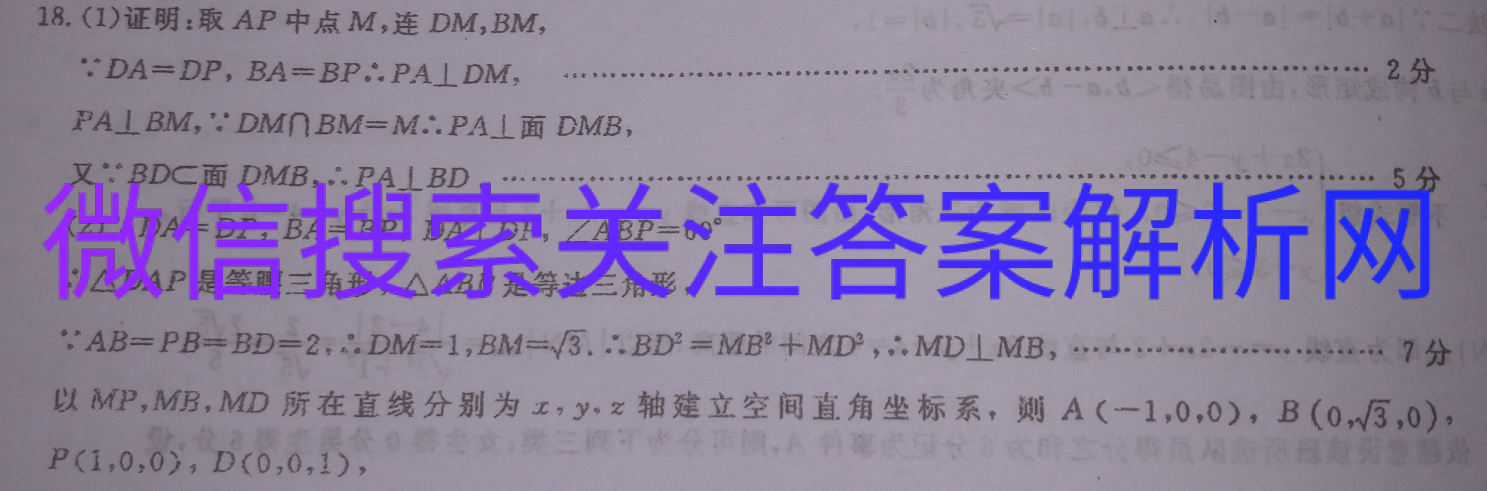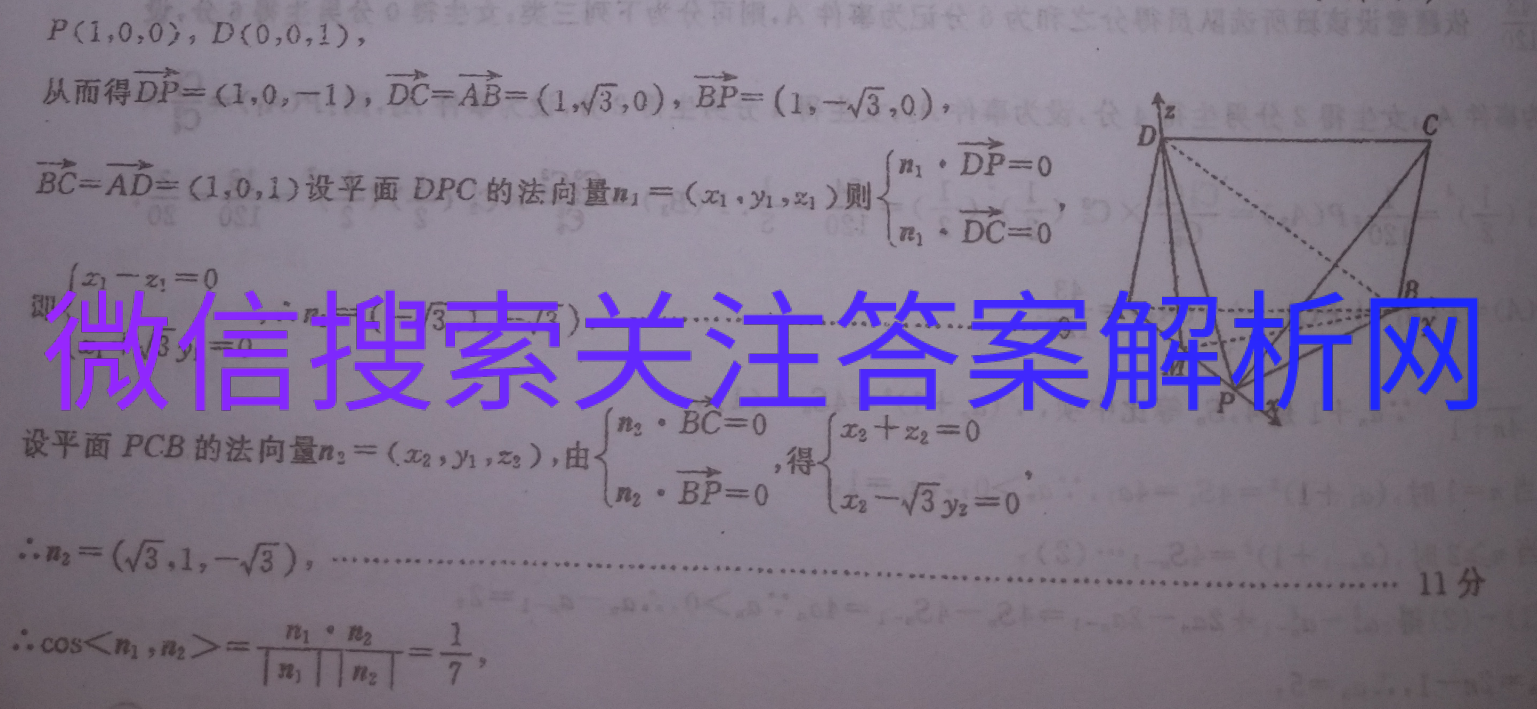# 全国100所名校最新高考模拟示范卷(一)(21·MNJ·N )

2021-03-07 14:5018.(1)证明:取AP中点M,连DM,BM,DA=DP,BA=BP。PA⊥DM2分FA⊥BM,∵DM∩BM=M∴PA⊥面DMB,又∵BDC面DMB,…PA⊥BD….5分(2)∵DA=DP,BA=BP,DA⊥DP,∠ABP=60余三比△DAP是等腰三角形,△ABP是等边三角形,AB=PB=BD=2,DM=1,BM=3.…∴BD3=MB2+MDP,∴MD⊥MB,………………7分以MP,MB,MD所在直线分别为x,yz轴建立空间直角坐标系,则A(-1,0,0),B(0,3,0),P(1,0,0),D(0,0,1),P(1,0,0),D(0,0,1)E=Ab=(.1)设平面DPC的法向量m一()),D0从而得DP=(1,0,-1),DC=AB=(1,3,0),BP=(1,-√3,0)7.DC=00即(-3,1,-√3)Ax1+/3y=0Mns. BC-设平面PCB的法向量n2=(x2,y1,z2),由0(x2+z2=0得B=01-3y2n2=(3,1,-√3),11分..cos a7故所求的三面角的正弦值为√一一49,更多内容，请微信搜索关注。